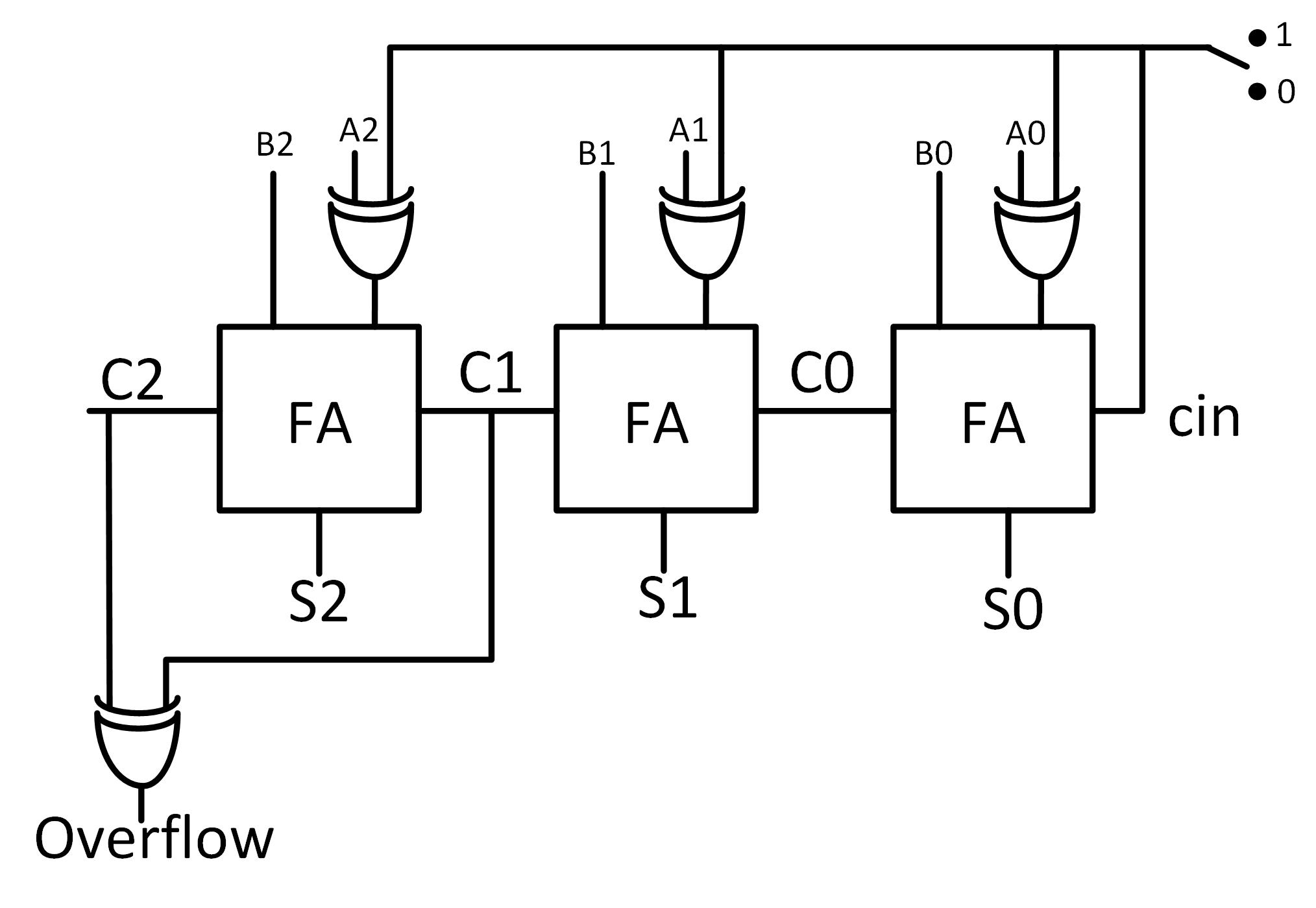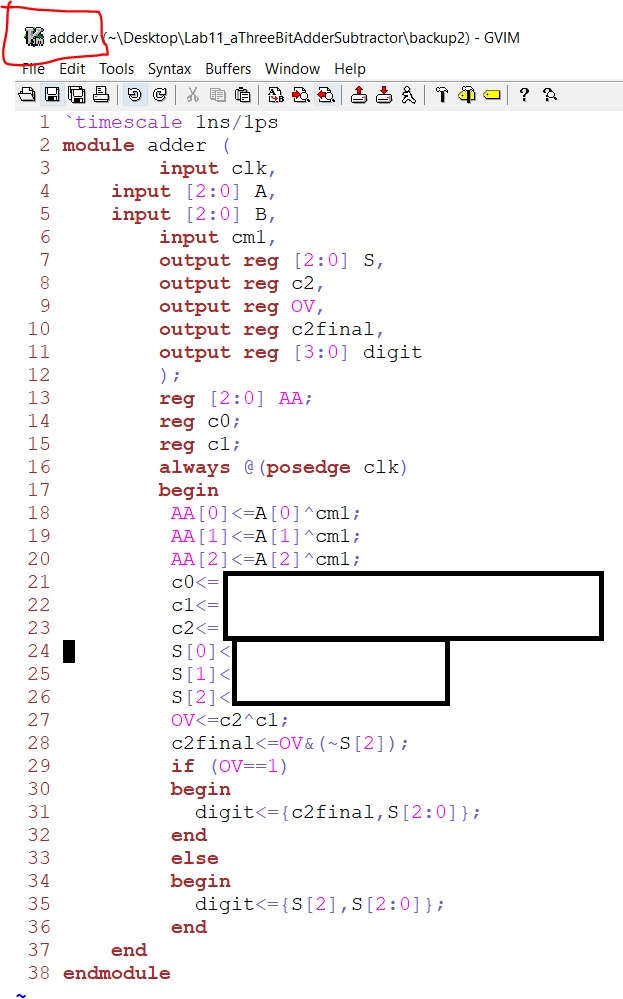Tutorial 3  A 3-bit Adder/Subtractor for 2's Complement Signed Binary Numbers

Outcome of this lab:
1. An ability to program an advanced sequential logic using Verilog Hardware Description Language.
2. An ability to buid a 3-bit adder/subtractor in 2's complement, and display the result in decimal.
3. An ability to verify the logic using FPGA. We will use the Basys3 FPGA board.

Instructions:
Use the updated constraint file here.

1, Understand the 3-bit adder/subtractor in 2's complement:signed binary numbers:
When the control bit is switched to '1', A XOR 1 = A not, so A is inverted, and plus 1 (at cin), which is exactly the 2's complement of A, the system will perform 'B+(-A)', which is 'B-A'; when the control bit is switched to '0', A XOR 0 =  A, and cin=0, the system will perform 'B+A'.

Overflow:
As the definition of 'overflow', if you have negtive + negative = positive, or positive + positive = negative, then the result of the addition is out of range, you need an extra bit to represent the result. The overflow indicator will show you when do you need to count an extra bit.

Your code should be able to tell FPGA what is the extra bit in order to display the correct corresponding decimal number.

Step 1:
Use switches as the 3-bit inputs, use 'leds' to show the binary results.

Step 2:
Use switches as the 3-bit inputs, use seven-segment displays to show the decimal result, make sure have the 'minus' sign in front of the decimal number if the result is negative.

The verification should be recorded in videos in your html report.

Part of the verilog code is given below. I designed three verilog modules: the adder module, the display module, and the 'top' module. The 'top' module is actually the testbench, which is easy. The 'adder' module is the calculator to deliver the final 4-bit result. The display module will take the result from the 'adder' module and display the results in the LEDs and the SSD.